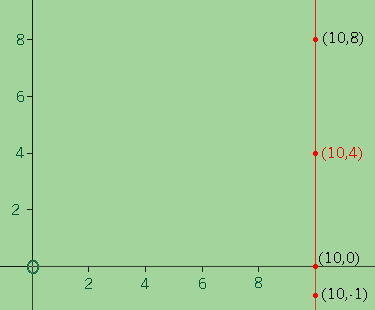SEARCH HOMEMath Central Quandaries & QueriesQuestion from Isabelle, a student: Hi! My question is this: Write the equation of each line described: A vertical line passing through (10,4) I would really appreciate your help! Thanks, IsabelleHi Isabelle,

I drew a diagram and identified a few more points on the line.Every point on the line has its x-coordinate equal to 10. Also every point in the plane with x-coordinate equal to 10 is on the line. Thus the points on the line are completely described by the statement "the x-coordinate is equal to 10". Said as an equation this is

$x = 10.$

PennyMath Central is supported by the University of Regina and The Pacific Institute for the Mathematical Sciences.